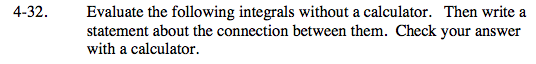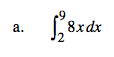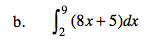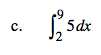### Home > CALC > Chapter Ch4 > Lesson 4.1.3 > Problem4-32

4-32.Sketch a graph. y = 8x is a linear function, and the area between x = 2 and x = 9 is trapezoidal.What effect does the +5 have on the bases of the trapezoid?Notice that the the trapezoid below the function being integrated in part (b) and above the function being integrated here is equivalent to the trapezoid you found in part (a).